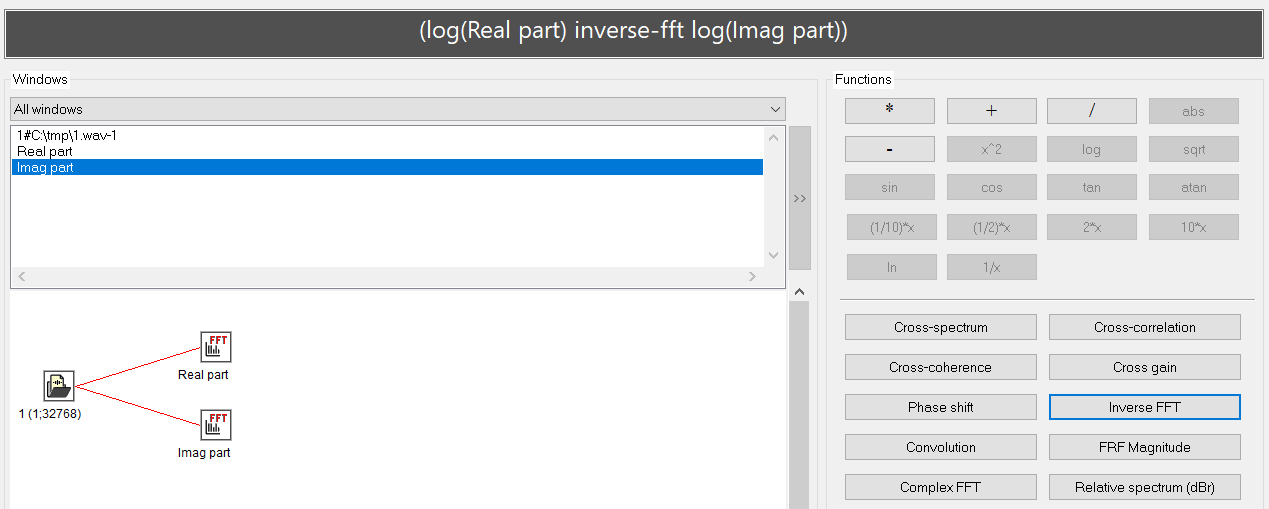By selecting “System control/Signal calculator” option from the main menu or pressing the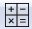toolbar button, you can start one of the most powerful tools in SIGVIEW, the Signal Calculator.

Just as a standard calculator is used for calculating expressions with numbers, SIGVIEWs calculator can be used to combine signals or instruments in different arithmetic or signal analysis expressions. You can use it, for example, to add/subtract two signals or spectra, or to perform different cross-spectral calculations.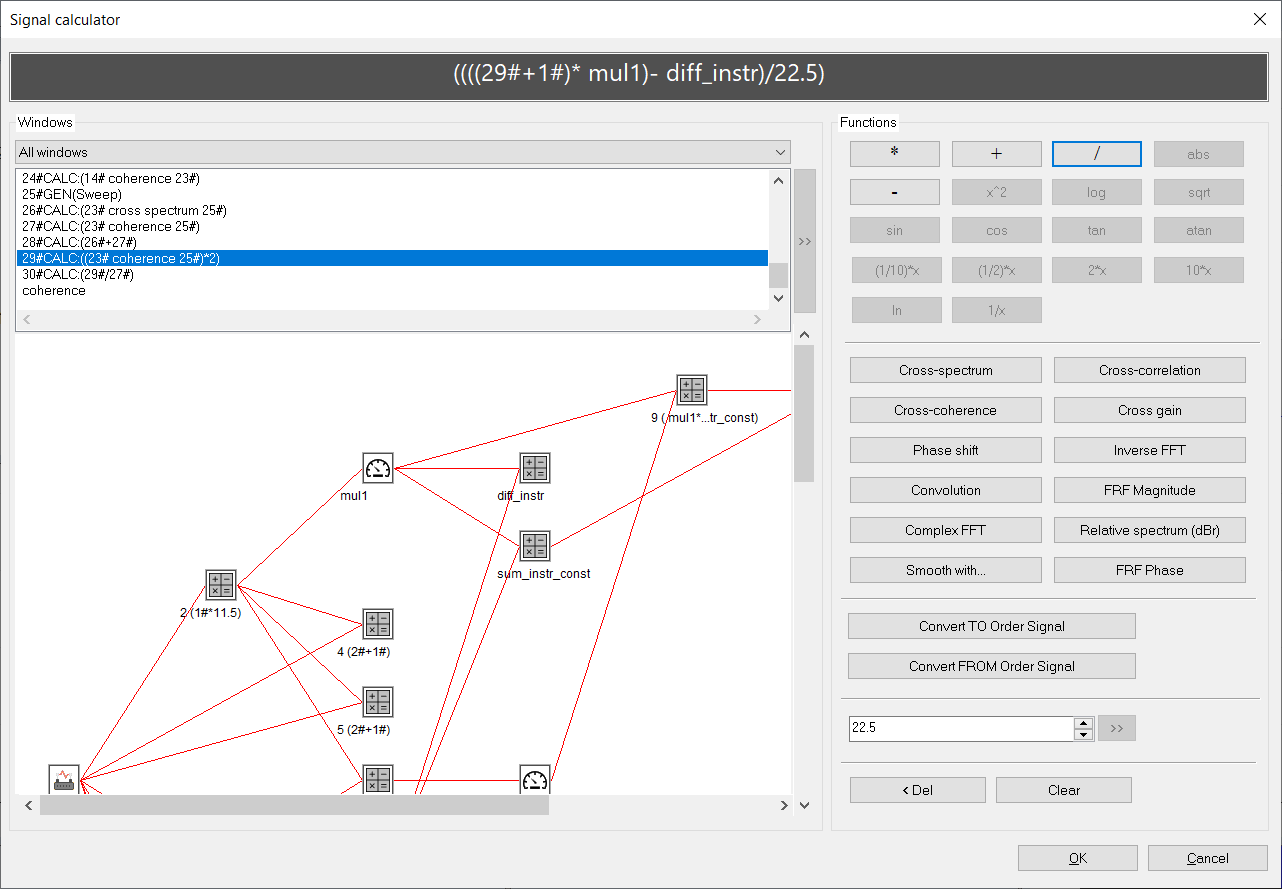Usage

A new calculator expression is created by combining existing windows and available functions or operations. The grey box on the top of the dialog will always contain the current expression.

On the left side of the dialog, the names of all existing signal or instrument windows (loaded signals, FFT results, etc.) in your SIGVIEW workspace will be displayed. You can filter this list to display all windows, only instruments, or only signal-like windows (sequences of values).

You can add signals/instruments in the expression by double-clicking their names or selecting them from the list and pressing the ‘>> button.

As an alternative to choosing windows from the list, you can also do it from the graphical display: Below the windows list, you will see a graphical display of your workspace, just as in Control Window. By simply clicking on a window icon, the corresponding window will be added to the expression. If a certain window is not suitable to be added to the current position in the expression, your mouse cursor will change to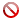and you will not be able to add that window to the expression.

Windows with standard titles and numerical identifiers (e.g. "17#FFT:..."), will be displayed in the expression only with their identifier and the hashtag character, e.g. "17#". Windows with custom title (e.g. "Spectrum1") will be displayed with their full title, e.g. "diff_instr". You can change the window title by using a corresponding option from its context menu or from the main menu.

At the right side of this dialog, various buttons with all available calculator functions are available. Only functions which are available in the given context will be enabled. For example, only after you add a signal window to your expression, binary function buttons like "+", "-", "/"... will be enabled.

Important note: Longer arithmetic expressions will be calculated in the order listed in the expression field and not according to the mathematical operator precedence. For example, the expression sqrt(A) + log(B) * C will be calculated as (sqrt(A) + log(B)) * C. The expression A+B*C+D+E*F will be calculated as ((((A+B)*C)+D)+E)*F.

For example, to subtract two signals, simply select the name of the first one, press the >> button to add it to the expression, then press the - button, and finally add the second signal to your expression.

When you create the complete calculator expression, click OK to create the result signal or instrument window. It will be linked to all windows included in the expression and will recalculate and redraw each time they change. If one of the windows used in the expression is deleted, the calculator window will be frozen and will retain its last values.

If only simple arithmetic binary functions (*, /, +, -) are used, you can freely mix instrument and signal windows in the expression. If only instruments have been used in the expression, the result will also be an instrument window.

If you include two signals of different lengths in a binary operation (for example, you subtract one signal from another one), SIGVIEW will use only a part of the longer signal (starting from its first visible sample) which has the length of the shorter signal.

### Functions

##### Unary functions (can be applied to a one signal or instrument window)

• sqrt (square root)
• log (log10)
• ln (loge)
• x^2 (squared)
• abs (absolute value)
• 2* (multiply with 2)
• 10* (multiply with 10)
• (1/2)* (multiply with 1/2 i.e. divide with 2)
• (1/10)* (multiply with 1/10 i.e. divide with 10)
• 1/x (1.0 divided by the values of a signal or instrument)
• sin, cos, tan, atan: trigonometric functions

##### Order Analysis functions [PRO version only]

For further information about Order Analysis functions, see Order Analysis chapter.

##### Numeric constant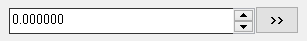By using a numeric field at the bottom of a function block, you can freely enter numeric constants and use them in the expression. Those can be used at the same positions in the expression where an instrument window could be used as well.

##### Clear & Delete functions

By using a 'Clear' button, you can clear the whole expression.

By using a '< Delete' button, you can delete the last expression entry.

### Examples

Below are some examples of calculator expressions:

1. Simple arithmetic operations on spectra

You have loaded two signals, calculated their spectra and you have the following workspace: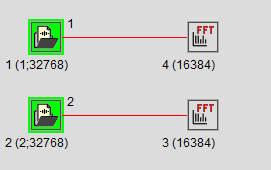Now, you would like to subtract two calculated spectra to get some information about their differences. To do so, create the following expression in the Signal calculator: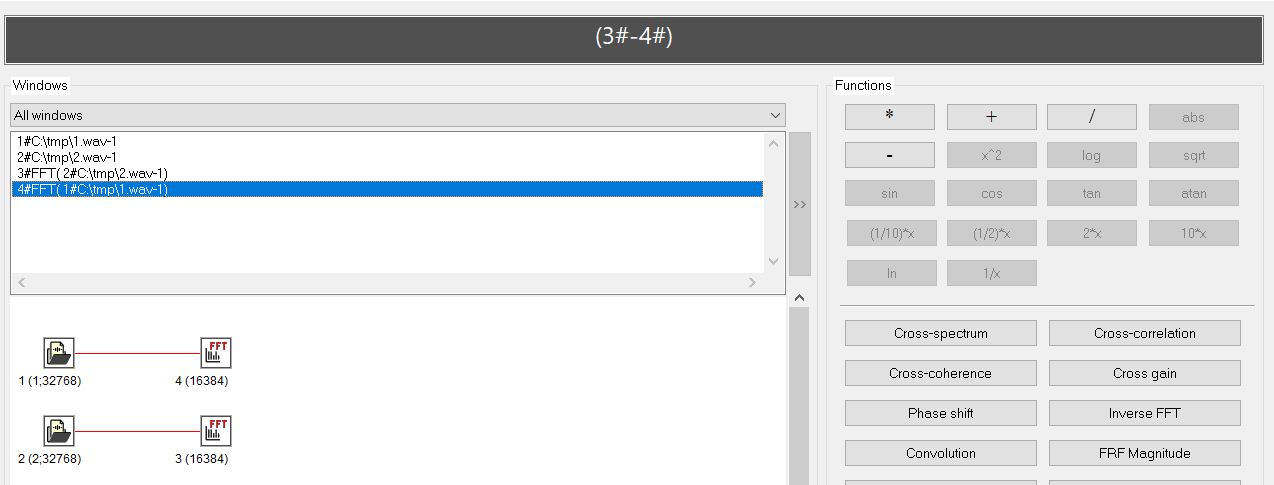The resulting window will contain the requested difference between spectra.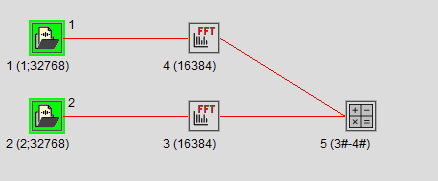2. Combining instruments and signals in one expression.

You would like to subtract the mean value of a signal from all signal values (normalisation). Firstly, you would calculate signal mean by using the appropriate instrument: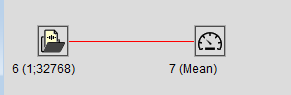Then, you would create the following expression in the Signal calculator: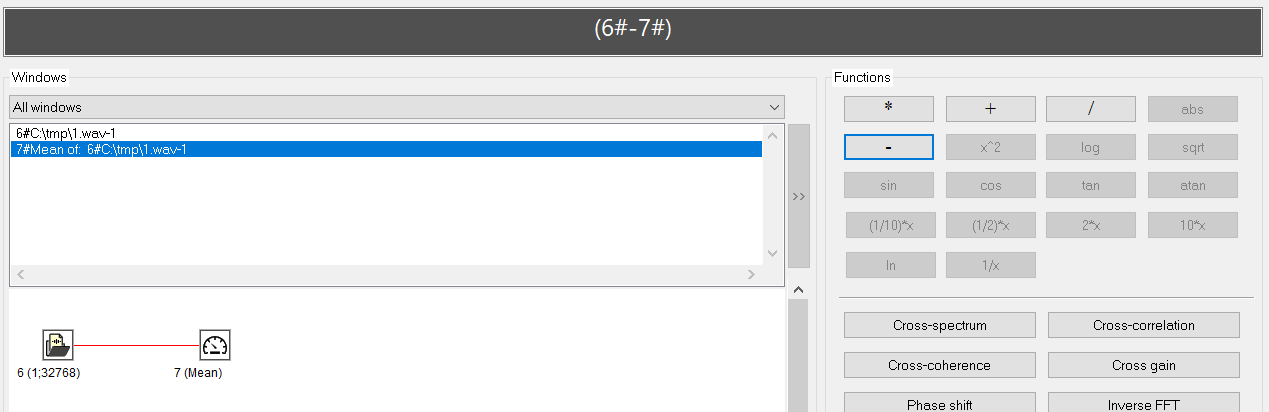The result will be a new signal calculated as requested: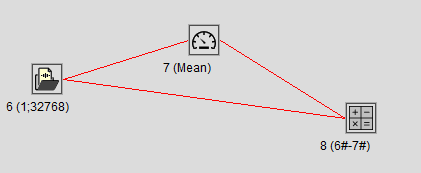2. Instrument-only expressions

You can also create expressions containing only instrument windows. The result of such expressions will also be a new instrument window.

In this example, we calculate the RMS of two signals and then create a new instrument containing their difference: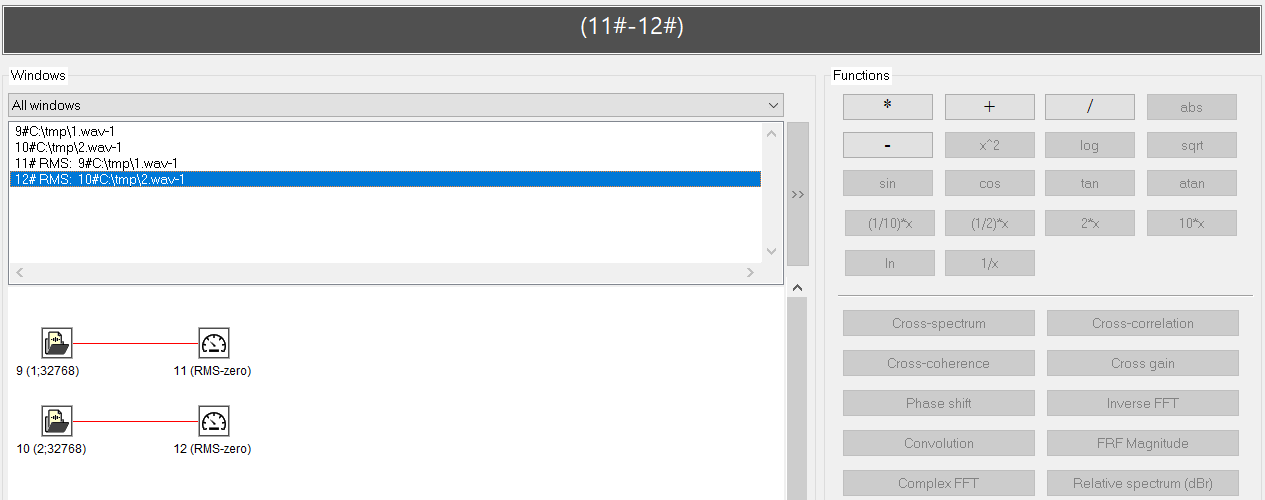The result will be a new instrument window: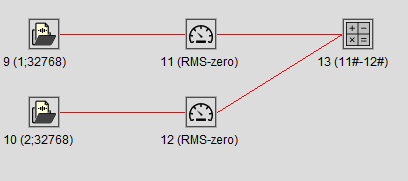3. Complex expressions.

There are practically no limitations for creating calculator expressions. Here are some examples:

f = A^2 + abs(B) - B^2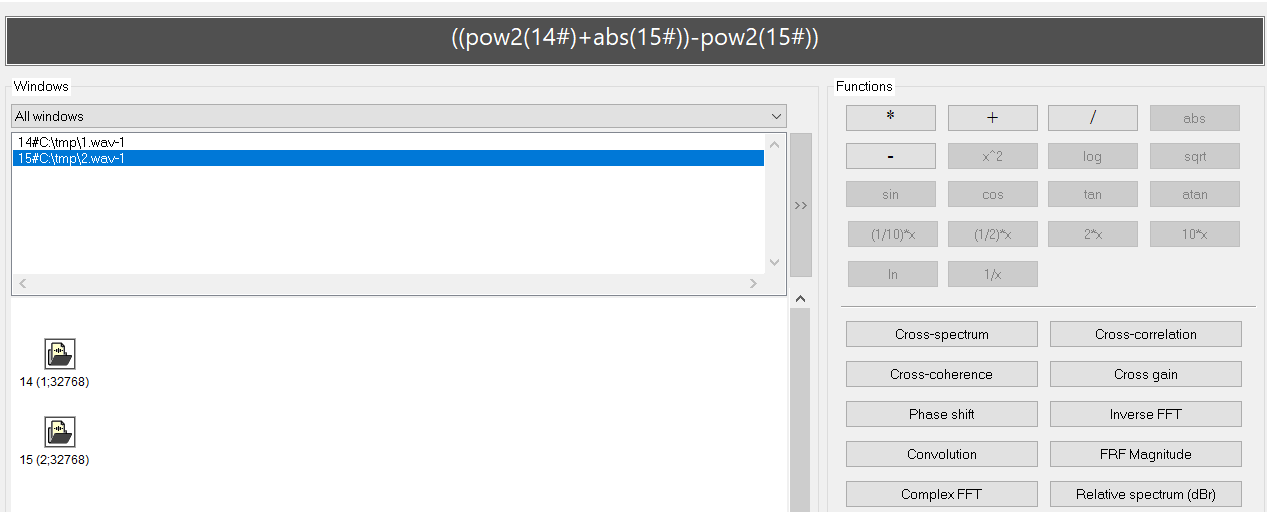Subtract spectrum of one signal from its cross spectrum with another signal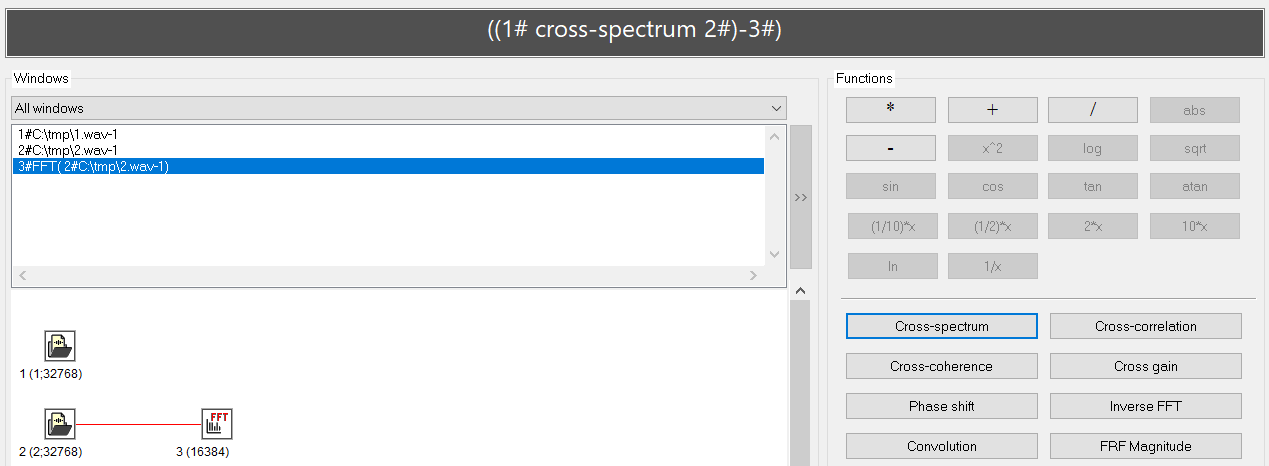Calculate log-values of both real and image spectrum and then perform inverse FFT